## Stefan’s Law

This law states that the total heat energy emitted by a perfect black body per second per unit area is directly proportional to the fourth power of the absolute temperature of its surface. Thus,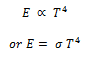If H is the rate of radiant energy emitted by a black body of surface area A, then the Stefan- Boltzmann law takes the form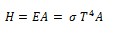Here σ is a universal constant called Stefan Boltzmann constant.

In SI units, σ = 5.67 × 10-8 J s-1 m-2 K-4

In CGS units, σ = 5.67 × 10-5 erg s-1 cm-2 k-4

The net loss of energy by the black body per unit area is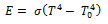If the body is not a perfect black body and has emissivity ε, then above relations get modified as follows: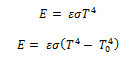## Wien’s displacement law

Wien’s displacement law states that the wavelength (λm) corresponding to which the energy emitted by a black body is maximum is inversely proportional to its absolute temperature (T).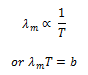Where b is Wien’s constant. Its value is 2.9 × 10 -3 mK.